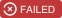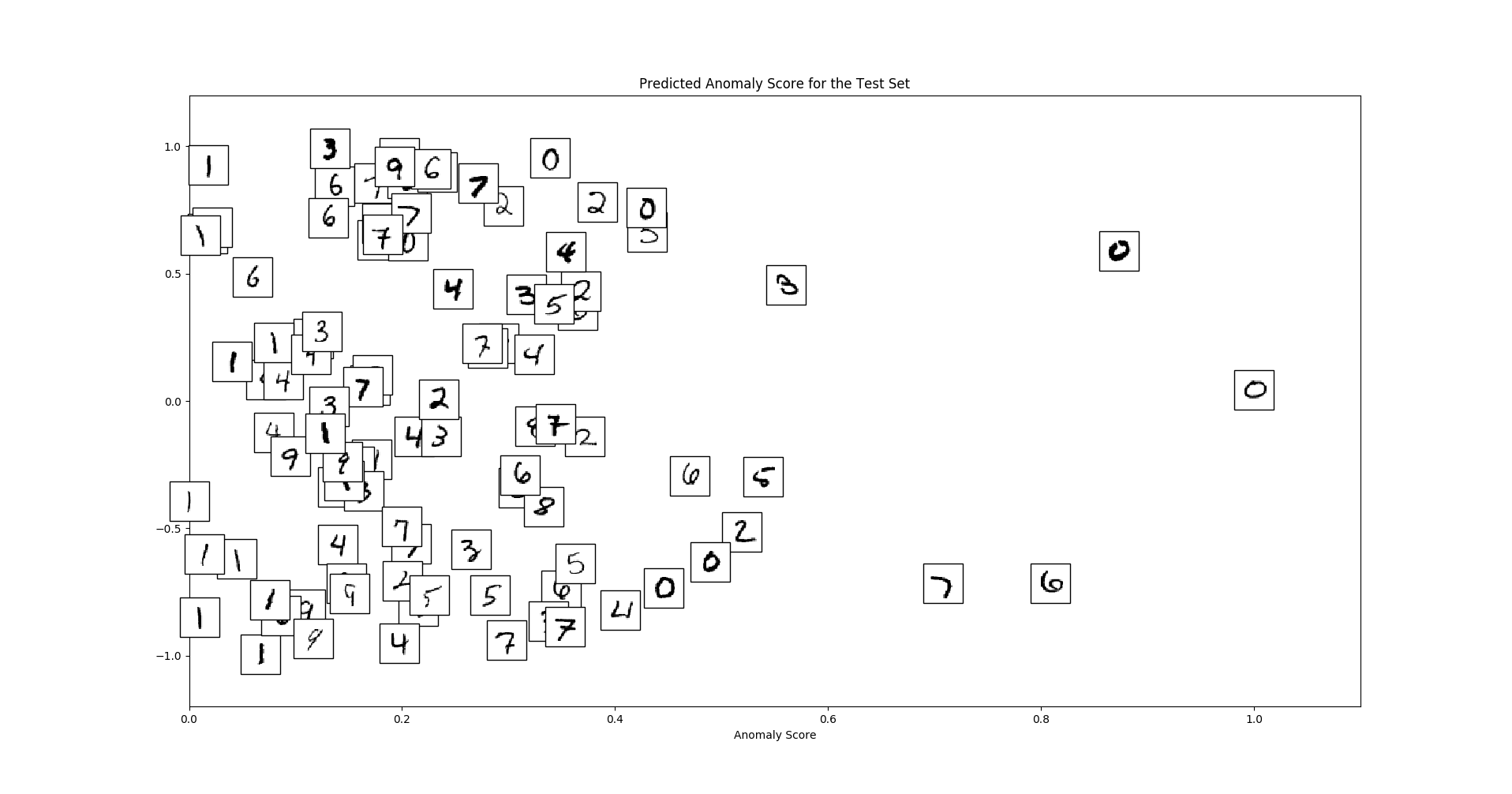# Anomaly Detection on Time Series: An Evaluation of Deep Learning Methods.The goal of this repository is to provide a benchmarking pipeline for anomaly detection on time series data for multiple state-of-the-art deep learning methods.

## Implemented Algorithms

Name Paper
LSTM-AD Long short term memory networks for anomaly detection in time series, ESANN 2015
LSTM-ED LSTM-based encoder-decoder for multi-sensor anomaly detection, ICML 2016
Autoencoder Outlier detection using replicator neural networks, DaWaK 2002
Donut Unsupervised Anomaly Detection via Variational Auto-Encoder for Seasonal KPIs in Web Applications, WWW 2018
REBM Deep structured energy based models for anomaly detection, ICML 2016
DAGMM Deep autoencoding gaussian mixture model for unsupervised anomaly detection, ICLR 2018
LSTM-DAGMM Extension of DAGMM using an LSTM-Autoencoder instead of a Neural Network Autoencoder

## Usage

```git clone git://github.com/KDD-OpenSource/DeepADoTS.git
virtualenv venv -p /usr/bin/python3
source venv/bin/activate
pip install -r requirements.txt
python3 main.py
```

## Example

We follow the scikit-learn API by offering the interface methods `fit(X)` and `predict(X)`. The former estimates the data distribution in an unsupervised way while the latter returns an anomaly score for each instance - the higher, the more certain is the model that the instance is an anomaly. To compare the performance of methods, we use the ROC AUC value.

We use MNIST to demonstrate the usage of a model since it is already available in TensorFlow and does not require downloading external data (even though the data has no temporal aspect).

```import pandas as pd
import tensorflow as tf
from sklearn.metrics import roc_auc_score

from src.algorithms import AutoEncoder
from src.datasets import Dataset

class MNIST(Dataset):
"""0 is the outlier class. The training set is free of outliers."""

def __init__(self, seed):
super().__init__(name="MNIST", file_name='')  # We do not need to load data from a file
self.seed = seed

def load(self):
# 0 is the outlier, all other digits are normal
OUTLIER_CLASS = 0
mnist = tf.keras.datasets.mnist
(x_train, y_train), (x_test, y_test) = mnist.load_data()
# Label outliers with 1 and normal digits with 0
y_train, y_test = (y_train == OUTLIER_CLASS), (y_test == OUTLIER_CLASS)
x_train = x_train[~y_train]  # Remove outliers from the training set
x_train, x_test = x_train / 255, x_test / 255
x_train, x_test = x_train.reshape(-1, 784), x_test.reshape(-1, 784)
self._data = tuple(pd.DataFrame(data=data) for data in [x_train, y_train, x_test, y_test])

x_train, y_train, x_test, y_test = MNIST(seed=0).data()
# Use fewer instances for demonstration purposes
x_train, y_train = x_train[:1000], y_train[:1000]
x_test, y_test = x_test[:100], y_test[:100]

model = AutoEncoder(sequence_length=1, num_epochs=40, hidden_size=10, lr=1e-4)
model.fit(x_train)

error = model.predict(x_test)
print(roc_auc_score(y_test, error))  # e.g. 0.8614
```

We can visualize the samples with respective error values as follows

```import numpy as np
import matplotlib.pyplot as plt
from matplotlib import offsetbox

"""Borrowed from https://github.com/scikit-learn/scikit-learn/blob/master/examples/manifold/plot_lle_digits.py#L44"""
error = (error - error.min()) / (error.max() - error.min())  # Normalize error
x_test = x_test.values
y_random = np.random.rand(len(x_test)) * 2 - 1
plt.figure(figsize=(20, 10))
ax = plt.subplot(111)
if hasattr(offsetbox, 'AnnotationBbox'):
shown_images = np.array([[1., 1.]])
for i in range(len(x_test)):
X_instance = [error[i], y_random[i]]
dist = np.sum((X_instance - shown_images) ** 2, 1)
if np.min(dist) < 4e-5:
# don't show points that are too close
continue
shown_images = np.r_[shown_images, [X_instance]]
imagebox = offsetbox.AnnotationBbox(offsetbox.OffsetImage(x_test[i].reshape(28, 28), cmap=plt.cm.gray_r), X_instance)
ax.add_artist(imagebox)
plt.xlim((0, 1.1))
plt.ylim((-1.2, 1.2))
plt.xlabel("Anomaly Score")
plt.title("Predicted Anomaly Score for the Test Set")
plt.show()
```

Which creates a plot like thisWe can see that global outliers (zeros) and local outliers (strangely written digits) receive high anomaly scores.

## Deployment

• `docker build -t deep-adots .`
• `docker run -ti deep-adots /bin/bash -c "python3.6 /repo/main.py"`

Team:

Supervisors:

## Credits

Get A Weekly Email With Trending Projects For These Topics
No Spam. Unsubscribe easily at any time.
python (54,487
deep-learning (3,987
pytorch (2,381
tensorflow (2,159
time-series (244
timeseries (103
anomaly-detection (92